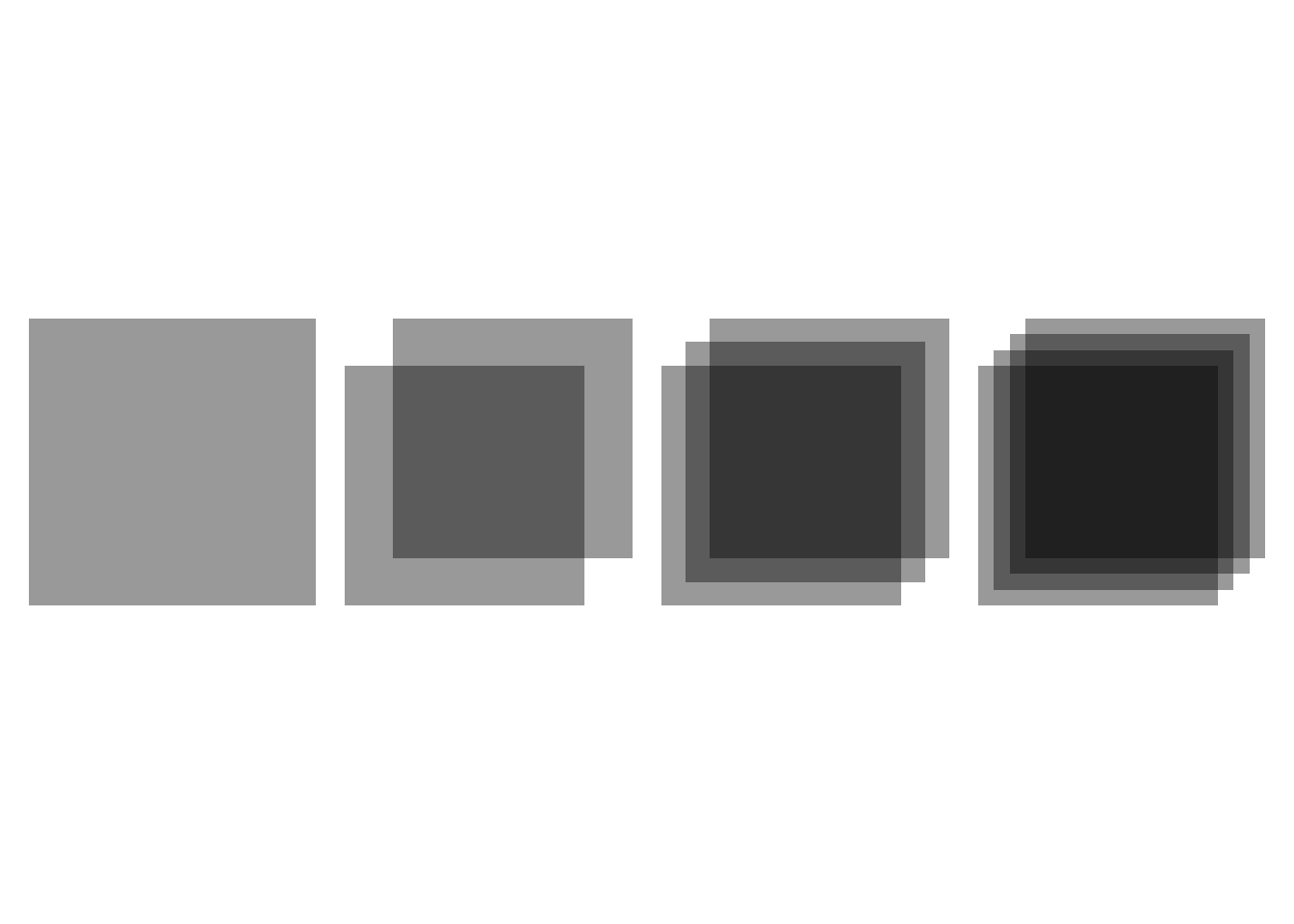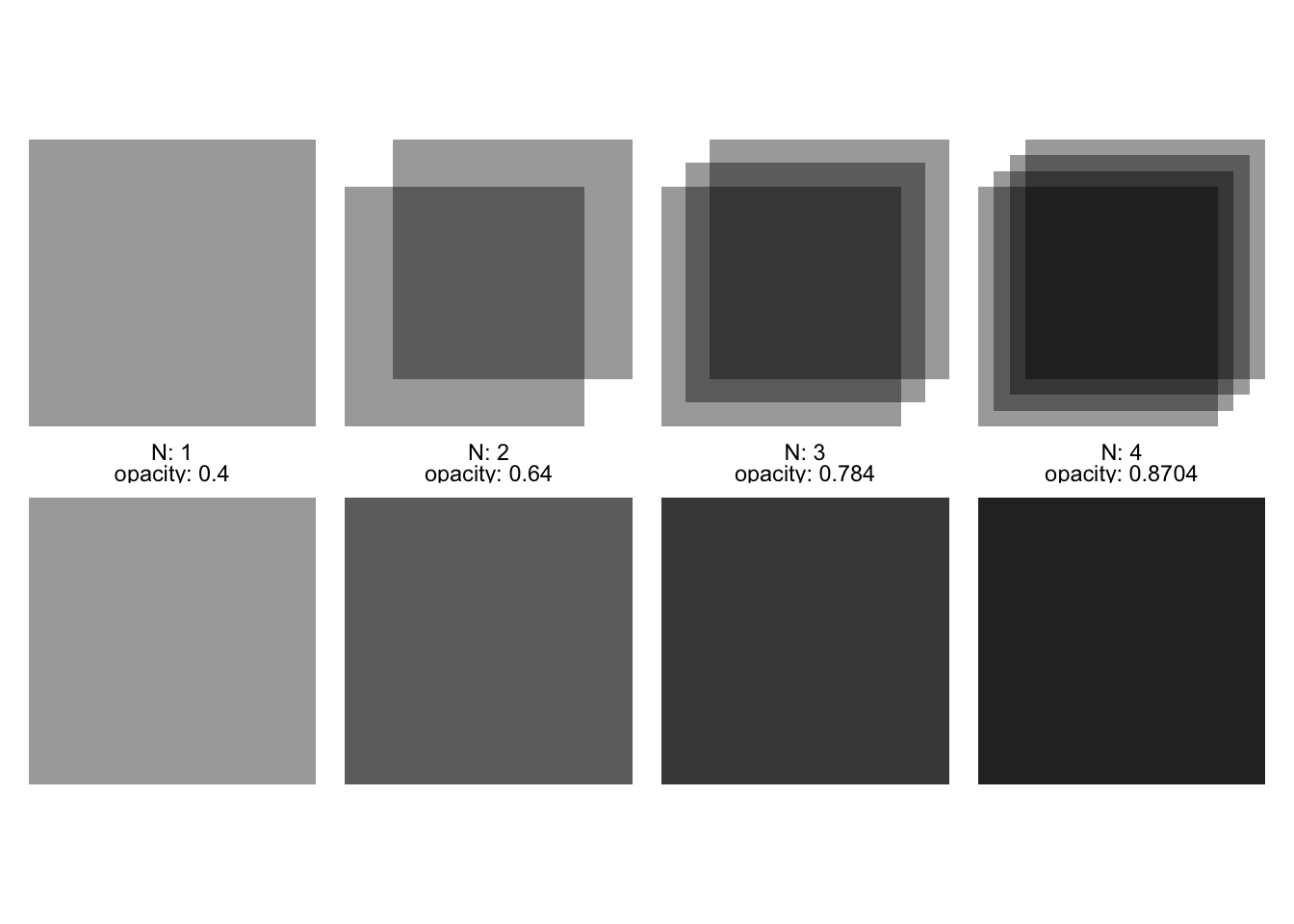# My mental model of how alpha works is wrong

## My mental model of alpha when plotting

When plotting overlapping elements with a defined alpha (opacity), I had always thought about it as an additive process with results clamped to not exceed 1.

Thus, without every bothering to check, my mental model was: three overlapping black rectangles, each with an opacity of 0.4, would result in a black rectangle since their combined opacity is 1.2, and this is clamped the maximum possible value of 1.

My mental model is wrong.

## What actually happens with overlapping alpha

In each sub-plot, I’ve plotted the overlap of a number of rectangles, each with `opacity` of 0.4.

The combined opacity increases with each overlap but doesn’t quite reach an opacity of 1.

``````#~~~~~~~~~~~~~~~~~~~~~~~~~~~~~~~~~~~~~~~~~~~~~~~~~~~~~~~~~~~~~~~~~~~~~~~~~~~~~~
# Simple function to create a plot with N overlapping rectangles with
# each having an alpha of 0.4
#~~~~~~~~~~~~~~~~~~~~~~~~~~~~~~~~~~~~~~~~~~~~~~~~~~~~~~~~~~~~~~~~~~~~~~~~~~~~~~
stack_alpha <- function(N) {
plot_df <- tibble(
x       = seq(0.45, 0.55, length.out = N),
y       = seq(0.45, 0.55, length.out = N),
opacity = 0.4
)

ggplot(plot_df) +
geom_tile(aes(x, y, alpha = I(opacity)), fill = 'black',
width=0.5, height = 0.5, colour = NA) +
theme_void() +
coord_equal()
}

plots <- lapply(1:4, stack_alpha)

patchwork::wrap_plots(plots, ncol = 4)``````## Correcting my thinking

Rather than talking about `opacity`, I should think in terms of `transmissivity` (i.e. `1-opacity`) - and transmissivity is a multiplicative property.

So, for a sequence of N layers with a transmissivity `t`, the transmissivity of the result is `t^N`, and thus the `opacity` is `(1 - t^N)`

The following plots show the original 4 plots from above, and below them plots of my estimation of the combined alpha using multiplicative transmissivity

N opacity transmissivity cumulative transmissivity cumulative alpha
1 0.4 0.6 0.6 1 - 0.6 = 0.4
2 0.4 0.6 0.6 * 0.6 = 0.36 1 - 0.36 = 0.64
3 0.4 0.6 0.6 * 0.6 * 0.6 = 0.216 1 - 0.216 = 0.784
4 0.4 0.6 0.6 ^ 4 = 0.1296 1 - 0.1296 = 0.8704
``````#~~~~~~~~~~~~~~~~~~~~~~~~~~~~~~~~~~~~~~~~~~~~~~~~~~~~~~~~~~~~~~~~~~~~~~~~~~~~~~
# Manually calculate the total transmissivity for 1-4 overlapping rectangles.
#~~~~~~~~~~~~~~~~~~~~~~~~~~~~~~~~~~~~~~~~~~~~~~~~~~~~~~~~~~~~~~~~~~~~~~~~~~~~~~
manually_stack_alpha <- function(N) {
plot_df <- tibble(
N = N,
x = 0.5,
y = 0.5,
tranmissivity = (1 - 0.4)^N,
opacity       = 1 - tranmissivity
)

ggplot(plot_df) +
geom_tile(aes(x, y, alpha = I(opacity)), fill = 'black',
width=0.5, height = 0.5, colour = NA) +
theme_void() +
coord_equal() +
facet_wrap(~N + opacity, labeller = label_both)
}

manual_plots <- lapply(1:4, manually_stack_alpha)

patchwork::wrap_plots(c(plots, manual_plots), ncol = 4)``````